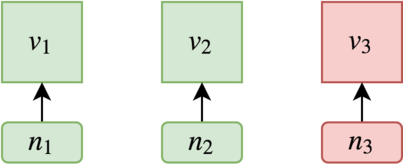# Task distribution over consensus nodes

Author: Alexey Vanin, alexey@nspcc.ru

Any computer system performs monitoring operation. It may send heartbeat messages, checksum queries, hash requests, etc. These operations are called tasks in this article. In centralized systems, tasks are performed by a certified node or a group of nodes. Decentralized systems can flexibly scale by delegating the execution of tasks among themselves, which is obviously efficient. However, the question arises — how to exactly distribute these tasks over selected nodes. We can solve this issue in two ways:

• nodes randomly select tasks for themselves
• nodes distribute tasks using consensus algorithms, e.g. dBFT

In this article, we discuss the second approach.

#### Byzantine fault tolerance task distribution

It is assumed there are tasks v and nodes n which are ready to work in the system. Each task and each node has a unique identifier. Thus, each node can choose tasks, using HRW , for execution by a predefined algorithm. Consensus on tasks distribution is the confirmation that all tasks are completed in the absence of technical failures.

Within the dBFT algorithm, consensus can be achieved even with up to 1/3 of compromised nodes in the network . An example of a system with n = v = 3 is considered:The system with 3 tasks and 3 nodes, where one is fraudulent

Tasks are uniformly distributed over nodes: if each node takes one task then, in the worst case, one task may remain non-executed. Redundancy is applied to ensure that all tasks are executed. Task v has to be executed by n/3 + 1nodes. In this case, a node task pool size is

In the considered case P(3,3) = 2

Whatever node is compromised, all tasks are still correctly executed.

The figure 1 shows — the function tends to a value of v/3, which means that for any each node task pool is one-third of all tasks. The system does not scale properly with load increase.

#### Reducing the task pool

For the system with n = 3, v = 4 size of task pool is P(3,4) = 2.3 3. A pool of tasks of each node can be reduced to 2:

Therefore, all tasks have been executed with a probability of 66 %. So, the task pool can be reduced, only need to do it with a certain precision.

For the research, a simulation model has been built, where experiments have been conducted with parameter v = 1000. For different n, there has been an attempt to find a task pool size, at which the probability of non-executed tasks is less than 0.00001. Model is available on github. Results are shown on figure 2:

It can be seen, experimental data from the model actually lie in the region between the maximum and minimum values of the task pool size. Therefore, as n grows, the required task pool size decreases. The difference between maximum task pool size and the experimental one can be approximated as a function R(x) (laid on the y1 axis) taking values from 0 to 1. Then the
task pool size can be calculated as

#### Further research

In this article, the probability of failures in network nodes is not considered and all experiments have been performed for the worst-case scenario with the number of compromised nodes is at its maximum. Obviously, the probability of compromising 33 out of 100 nodes is less than 1 out of 3 nodes. Therefore, it is potentially possible to determine the “compromise probability” function Q(x), and thus, calculate the pool size as P(n,v)⋅R(n)⋅Q(n). The behavior of the function R(x), when the number of compromised nodes is less than n/3,
has to be studied.

#### References

1. E. Zhang, A Byzantine Fault Tolerance Algorithm for Blockchain:
https://docs.neo.org/en-us/basic/consensus/whitepaper.html
2. Rendezvous (highest random weight) hashing:
https://en.wikipedia.org/wiki/Rendezvous_hashing
3. Simulation model repository: